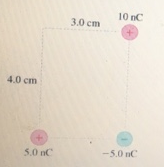# Problem: What is the magnitude of the force F on the 5.0 nC charge in the figure? What is the direction of the force F on the 5.0 nC charge in the figure?

###### FREE Expert Solution

From Coulomb's law:

$\overline{)\stackrel{\mathbf{⇀}}{\mathbf{F}}{\mathbf{=}}\frac{\mathbf{k}{\mathbf{q}}_{\mathbf{1}}{\mathbf{q}}_{\mathbf{2}}}{{\mathbf{r}}^{\mathbf{2}}}\stackrel{\mathbf{^}}{\mathbf{r}}}$

The angle between 5.0 nC and 10nC is obtained by:

θ = tan-1(4.0/3.0) = 53.1°

Force on 5nC due to 10nC:

$\begin{array}{rcl}{\mathbf{F}}_{\mathbf{1}}& \mathbf{=}& \frac{\mathbf{\left(}\mathbf{9}\mathbf{×}{\mathbf{10}}^{\mathbf{9}}\mathbf{\right)}\mathbf{\left(}\mathbf{5}\mathbf{×}{\mathbf{10}}^{\mathbf{-}\mathbf{9}}\mathbf{\right)}\mathbf{\left(}\mathbf{10}\mathbf{×}{\mathbf{10}}^{\mathbf{-}\mathbf{9}}\mathbf{\right)}}{{\mathbf{\left[}\sqrt{{\mathbf{\left(}\mathbf{4}\mathbf{.}\mathbf{0}\mathbf{×}\mathbf{10}\mathbf{-}\mathbf{2}\mathbf{\right)}}^{\mathbf{2}}\mathbf{+}{\left(3.0×10-2\right)}^{2}}\mathbf{\right]}}^{\mathbf{2}}}\mathbf{\left[}\mathbf{c}\mathbf{o}\mathbf{s}\mathbf{\left(}\mathbf{53}\mathbf{.}\mathbf{1}\mathbf{°}\mathbf{\right)}\mathbf{\left(}\mathbf{-}\stackrel{\mathbf{^}}{\mathbf{i}}\mathbf{\right)}\mathbf{+}\mathbf{s}\mathbf{i}\mathbf{n}\mathbf{\left(}\mathbf{53}\mathbf{.}\mathbf{1}\mathbf{°}\mathbf{\right)}\mathbf{\left(}\mathbf{-}\stackrel{\mathbf{^}}{\mathbf{j}}\mathbf{\right)}\mathbf{\right]}\end{array}$

96% (424 ratings)###### Problem DetailsWhat is the magnitude of the force F on the 5.0 nC charge in the figure?

What is the direction of the force F on the 5.0 nC charge in the figure?